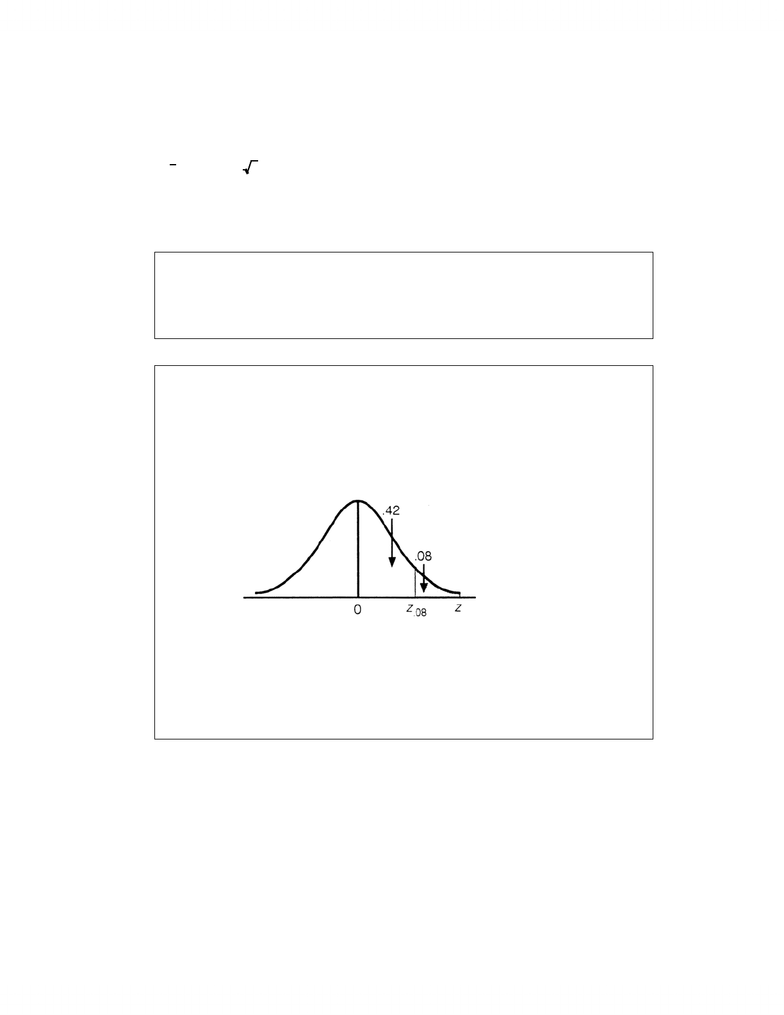# Chapter 10 Textbook Study Guide

32 views6 pages
School
Course
ProfessorChapter 10: Introduction to Estimation
10.1 Introduction
This chapter introduced estimation, a form of statistical inference. Make certain that you understand
the sampling distribution of the mean before proceeding.
At the completion of this chapter, you are expected to know the following:
1. Understand the fundamental concepts of estimation.
2. How to produce interval estimates of the population mean.
3. How to interpret interval estimates.
4. How to determine the sample size to estimate a population mean.
10.2 Concepts of Estimation
This section dealt with the fundamental concepts of estimation. You are expected to know the mean-
ing of the terms introduced in this chapter and understand why estimation is necessary in most practical
situations.
EXERCISES
10.1 What is the difference between an estimator and an estimate?
10.2 Define each of the following terms:
a) Unbiased estimator
b) Consistent estimator
10.3 Explain why
x
is an unbiased estimator of µ.
10.4 Explain why s2 is an unbiased estimator of σ2.
10.5 Explain why
x
is a consistent estimator of µ.
115
Unlock document

This preview shows pages 1-2 of the document.
Unlock all 6 pages and 3 million more documents.10.3 Estimating the Population Mean Value When
the Population Standard Deviation Is Known
We presented the technique to produce a interval estimate of a population mean when the
population variance is known. The interval estimator of µ is
x ±zα/2σ/n
It is assumed that the population variance is known. The confidence level is equal to 1 – α, and from this
value you need to be able to determine
z
α/2
. Other than plugging the numbers into the formula, no
other arithmetic skills are required.
Question: How can I tell when the population variance is known?
Answer: The question must state explicitly that the population variance σ2 is known,
and it must also specify the value.
Question: How do I find
z
α/2
?
Answer: The question will specify the confidence level 1 – α. From the confidence
level, determine a and divided it by 2. For example, if the confidence level is
.84, then since 1 – α = .84 it follows that α = .16 and α/2 = .08. To find
z
α/2 =
z
.08 , we need to find the value of z such that the area to its right is
.08.
Since Table 3 is designed to provide the areas between 0 and z, we need to
subtract .08 from .5 to produce .42. (Notice that we can get .42 more directly
by dividing the confidence level by 2.) Now in Table 3 find the area closest to
.42 (.4200). As you should be able to see, the area between 0 and 1.40 is
.4192 and the area between 0 and 1.41 is .4207. Since the latter area is closer
to .4200, we find z.08 = 1.41.
116
Unlock document

This preview shows pages 1-2 of the document.
Unlock all 6 pages and 3 million more documents.

# Get access

\$10 USD/m
Billed \$120 USD annually
Homework Help
Class Notes
Textbook Notes